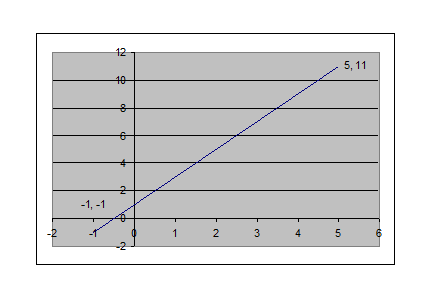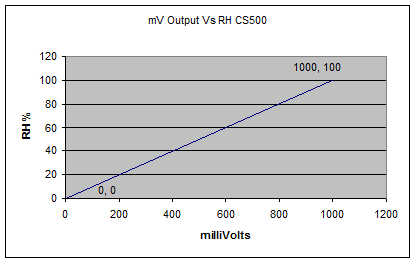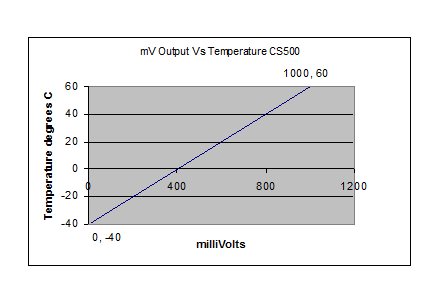Background

Many of the instructions in Campbell Scientific datalogger operating systems have parameters where a multiplier and an offset can be entered. This allows the programmer to scale the units of the measurement from the electrical parameter that was measured by the logger, into more applicable engineering units for the sensor in question.

The multiplier and offset are used when the relationship between the measured electrical output (say voltage) and the parameter being measured (say temperature) is linear. If the relationship between two parameters is linear, there is a straight line that can be drawn on a graph to describe this relationship.

The equation of this line will be Y = mX + b where m is the multiplier (or slope of the line) and b is the offset(or the y-intercept of the line). All straight lines can be represented mathematically in this way.

Mathematical ExampleCalculating the multiplier and offset from a straight line graph such as this one is straightforward.

Multiplier

To calculate the multiplier (slope) we use the equation

m = rise/run = (change in y)/(change in x) = (11- (-1))/(5- (-1))

m = 12/6 = 2

So our slope (multiplier) is 2.

This means that for every 1 unit we move along the x-axis, the value of y changes by 2.

Offset

To calculate the offset (y-intercept) we do the following:

The y-intercept occurs when the line crosses the vertical axis (y-axis).

This occurs when x = 0.

Looking at the graph, we can guess that the y-intercept (the value of y when x = 0) is 1.

To calculate this value, we use the coordinates of one of a nearby point on the line, in this case (-1,-1) and the slope of the line (2).

The equation used to calculate this value is:

b = multiplier x (0 – (x-value of the nearby point)) + (y-value of the nearby point)

b = 2 x (0-(-1)) + (-1)

b = (2 x 1) – 1

b = 2-1 = 1

So our offset is 1.

Relating this to the logger

Now, we have spoken in fairly generic mathematical terms and we’ve heard a lot about x and y. It’s time to relate this to the loggers and measurements of real values.

The x value is equivalent to the value that the logger measures. It might be a number representing the mV output of the sensor or it could be the number of counts on a pulse channel in the last interval.

The y value is equivalent to the solar radiation in kW/m2, the temperature in degrees, the wind speed in metres/second or the rainfall in mm.

Our multiplier and offset will describe the straight line that relates our logger measurement (x) to the parameter in question (y).

This will work out the same from any point on the line. Check by using the point (5,11).

b = 2 x (0-5) + 11

b = -10 + 11 = 1 (the same result).

Example

Calculating Multiplier and Offset for CS500 Temperature and Relative Humidity Output

CS500 specifications:

Temperature Output: 0-1000mV for -40 to +60 degrees C.

Relative Humidity Output: 0-1000mV for 0-100% RH.

If we plot these on graphs, they look like this:

Temperature.Now, we calculate the multiplier and offset.

Multiplier = rise/run = (60-(-40))/(1000-0) = 100/1000 = 0.1

Using the point (1000,100) and a multiplier of 0.1

Offset = multiplier x (0 – (x-value of the nearby point)) + (y-value of the nearby point)

Offset = 0.1 x (0-1000) + (60) = 0.1 x (-1000) + 60 = -100 + 60 = -40

We can see that this offset is correct as we have one of the points at (0,-40) which is the y intercept (at x=0).

Relative HumidityMultiplier = rise/run = (100-0)/(1000-0) = 100/1000 = 0.1

We can see that the offset is 0 because we have coordinates of the point at x=0 (0,0).

To check, we use the point (1000,100) and the multiplier = 0.1

Offset = multiplier x (0 – (x-value of the nearby point)) + (y-value of the nearby point)

Offset = 0.1 x (0-1000) + (100) = 0.1 x (-1000) + 100 = -100 + 100 = 0

Exercises

Calculate the multipliers and offsets that would be used for the following:

• Greenspan Pressure Sensor 0-5metre range with 0-2500mV output.
• Wind Direction Vane. 10k ohm potentiometer excited with 2000mV. Measurement range 0-355 degrees (5 degrees open)
• Battery Voltage monitor. 0-1.5V output for 10-13V input.
• What multiplier and offset would be used if the raw mV output of the CS500 temperature element was to be stored?